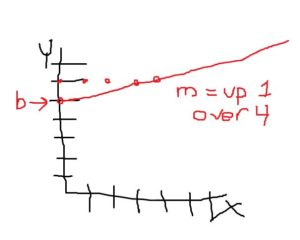# The confusion nomenclature of epidemiology and biostatistics

## This should be more simple.

Epidemiology and biostatistics are awash with synonyms and each institution has its own preferred nomenclature to describe the same general concepts. I started this page as a central place to document the various terms by concept. I’ll plan on revisiting and updating over time.

## Regression

### Fundamentals

You probably learned the fundamentals of regression in introductory algebra but may not realize it.  Remember drawing a graph from a slope-intercept equation? Draw a graph where Y is equal to 1/4x plus 5. (Here is the relevant Khan Academy Algebra I video about this.) You take the general equation:

Y = mx + b

…where Y is the y-axis, m is the slope of the line, and b is where the line crosses the y-axis. The equation you will write is:

Y=1/4x + 5

…and you will draw:This sounding familiar? When you do a linear regression, you do the same thing. Instead, you regress Y on X, or:

Y = β1x1 + β0

And fitting in the variables here, you want to figure out what a predicted cholesterol level will be for folks by a given age. You would regress cholesterol level on age:

Cholesterol level = β1*Age + β0

Here, x1 is the slope of the line for age and β0 is the intercept on the Y-axis, essentially the same as the b in Y=mx+b. When you run a regression in Stata, you type

`regress y x`

or here,

`regress cholesterol age`

Let’s say that Stata spits out something like:

```      Source |  xxxxxxxxxxxxxxxxxxxxxxxxxxxx
-------------+------------------------------
Model |  xxxxxxxxxxxxxxxxxxxxxxxxxxxx
Residual |  xxxxxxxxxxxxxxxxxxxxxxxxxxxx
-------------+------------------------------
Total |  xxxxxxxxxxxxxxxxxxxxxxxxxxxx

------------------------------------------------------------------------------
cholesterol|      coeff       se         t     P>|t|    [95% Conf. Interval]
-------------+----------------------------------------------------------------
age |     0.500     xxxxxxxx    xxxxx   0.000     0.4000      0.60000
_cons |     100       xxxxxxxx    xxxxx   0.000     90.000      110.000
------------------------------------------------------------------------------```

The βcoefficient for age is 0.5. The intercept, or β0 is 100. You would interpret this as cholesterol level = 0.5*age in years + 100. You could plot this using your Algebra 1 skills.

Cholesterol = 0.5*age + 100

Or you can substitute in actual numbers. What is the predicted cholesterol at age 50? Answer: 125.

If you want to make it more complex and add more variables to explain cholesterol level, it’s no longer a straight line on a graph, but the concept is the same. A multiple linear regression adds more X variables. You can figure out what a predicted cholesterol level will be for folks by age, sex, and BMI. You would regress cholesterol level on age, sex, and BMI. (You would code sex as 0 or 1, like female = 1 and male = 0.)

Y =  β1x1 + β2x2 + β3x3 + β0

Or,

Y =  β1*Age + β2*Sex + β3*BMI + β0

You get the idea.

### Names of Y and X

This is what irks me. There are so many synonyms for Y and X variables. Here is a chart that I’ll update over time with synonyms seen in the wild.

Y = x
Dependent Independent
Outcome Predictor
Covariate
Factor
Exposure variable
Explanatory variable